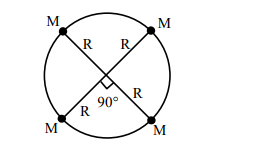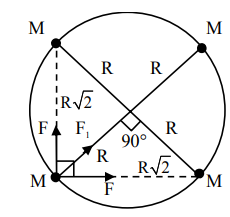# Four particles each of mass M, move along a circle of radius R under the action of their mutual gravitational attraction as shown in figure. The speed of each particle is :`
Question:

Four particles each of mass M, move along a circle of radius R under the action of their mutual gravitational attraction as shown in figure. The speed of each particle is :1. $\frac{1}{2} \sqrt{\frac{\mathrm{GM}}{\mathrm{R}(2 \sqrt{2}+1)}}$

2. $\frac{1}{2} \sqrt{\frac{\mathrm{GM}}{\mathrm{R}}(2 \sqrt{2}+1)}$

3. $\frac{1}{2} \sqrt{\frac{\mathrm{GM}}{\mathrm{R}}(2 \sqrt{2}-1)}$

4. $\sqrt{\frac{\mathrm{GM}}{\mathrm{R}}}$

Correct Option: , 2

Solution:$\mathrm{F}_{\mathrm{net}}=\frac{\mathrm{MV}^{2}}{\mathrm{R}}$

$\sqrt{2} \mathrm{~F}+\mathrm{F}_{1}=\frac{\mathrm{MV}^{2}}{\mathrm{R}}$

$\sqrt{2} \frac{\mathrm{GMM}}{(\sqrt{2} \mathrm{R})^{2}}+\frac{\mathrm{GMM}}{(2 \mathrm{R})^{2}}=\frac{\mathrm{MV}^{2}}{\mathrm{R}}$

$\frac{\mathrm{GM}}{\mathrm{R}}\left(\frac{1}{\sqrt{2}}+\frac{1}{4}\right)=\mathrm{V}^{2}$

$\frac{\mathrm{GM}}{\mathrm{R}}\left(\frac{4+\sqrt{2}}{4 \sqrt{2}}\right)=\mathrm{V}^{2}$

$\mathrm{V}=\sqrt{\frac{\mathrm{GM}(4+\sqrt{2})}{\mathrm{R} 4 \sqrt{2}}}$

$\mathrm{~V}=\frac{1}{2} \sqrt{\frac{\mathrm{GM}(2 \sqrt{2}+1)}{\mathrm{R}}}$

Option (2)